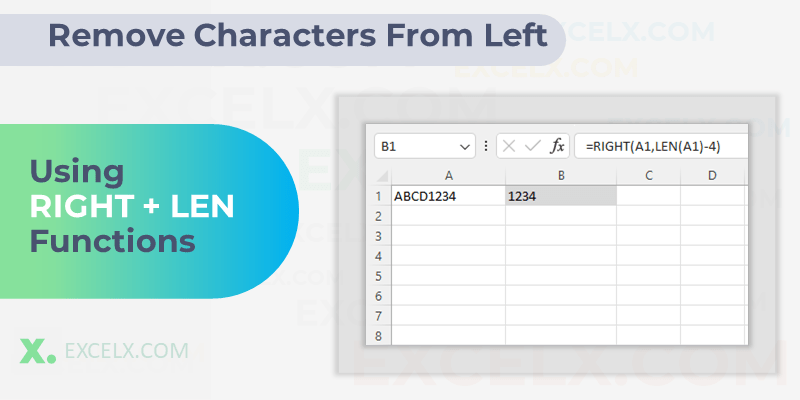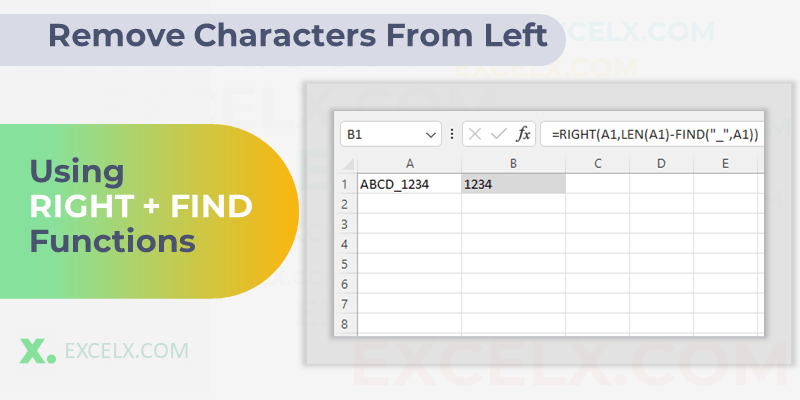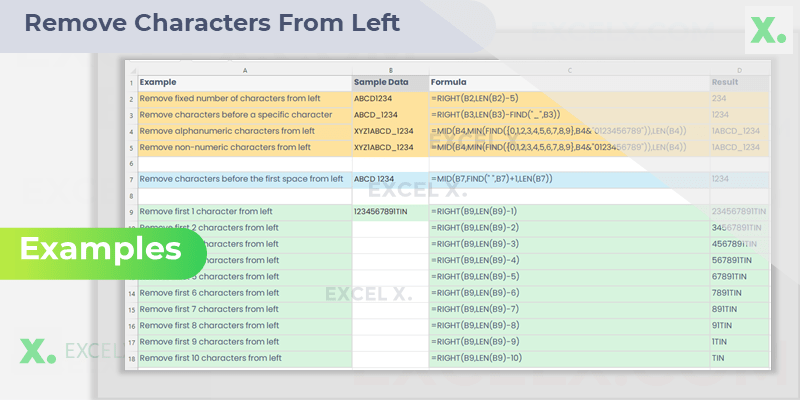When we process the data in Microsoft Excel, you’ll often find yourself in a situation where you need to remove certain characters from the left ( or beginning) of the text in a Cell. This can be especially useful when you need to clean up data that may have unwanted or unnecessary prefixes. Learn how to remove characters from the left side of a string using Excel formulas such as RIGHT, LEN, and FIND. Improve your data cleaning and enhance productivity with these handy Excel tips.

## Excel Formula to Remove Characters From Left

Excel offers a variety of formulas to remove characters from Left (beginning), the most commonly used are the RIGHT, LEN, and FIND functions. In this post, we’ll walk you through the steps of removing characters from the left using Excel formulas.

### Remove using RIGHT and LEN Functions

The RIGHT and LEN functions are two powerful functions in Excel. The RIGHT function allows you to extract a certain number of characters from the right end of a text string, while the LEN function returns the length of a text string in terms of the number of characters.Here’s an example of how you can use the RIGHT and LEN functions together to remove the first two characters from a cell:

Suppose you have “ABCD1234” in cell A1, and you want to remove the “ABCD” prefix from the string.

Enter the following formula into another cell:

```=RIGHT(A1,LEN(A1)-4)
```

The LEN function calculates the length of the string (8 characters in this case), and then we subtract 4 (Length of “ABCD”) from it. The RIGHT function then takes the resulting number (4) and extracts that many characters from the right end of the text string. The result will be “1234”.

### Remove using FIND Function

Another way to remove characters from the left of a string is by using the FIND function in combination with RIGHT and LEN. This is particularly useful when the number of characters to remove is not fixed, but depends on the position of a specific character.For example, if you want to remove everything before (and including) an underscore in the string “ABCD_1234” in cell A1, you can use the following formula:

```=RIGHT(A1,LEN(A1)-FIND("_",A1))
```

Here, the FIND function identifies the position of the underscore (5 in this case). The LEN function determines the total length of the string (9 characters), and we subtract the position of the underscore. The RIGHT function then extracts the resulting number of characters (5) from the right end of the text string. The result will be “1234”.

### Remove using REPLACE Function

The REPLACE function is a versatile function that can be used to replace characters, words, or even entire strings of text. To use the REPLACE function to remove characters from the left of a cell.For example, if you want to remove first 4 Characters from the String (“ABCD1234”) in cell A1, you can use the following formula:

```=REPLACE(A1, 1, 4, "")
```

Here, the REPLACE function Replace an Empty String (“”) in place of the first 4 characters and results remaining characters “1234”.

### Which method should you use?

In conclusion, while Excel does not provide a dedicated function to remove characters from the left, using combinations of existing functions can help you accomplish the same task effectively. The method that you use to remove characters from the left of a cell will depend on your specific needs.

You can use FIND Function when you wants to find a Character and wants to remove all the Characters before that specific Character.

If you only need to remove a few fixed number of characters, then RIGTH with MID function or REPLACE function are good options. If you need to remove a larger number of characters, the RIGHT function or the MID function may be a better choice.

### Examples

In the upcoming examples, we’ll demonstrate how you can manipulate text strings in Microsoft Excel by removing a specified number of characters from the left. This ability is crucial in many instances, especially when cleaning and organizing data for analysis.Example Formula
Remove fixed number of characters from left =RIGHT(A1,LEN(A1)-N)
Remove characters before a specific character =RIGHT(A1,LEN(A1)-FIND(“_”,A1))
Remove alphanumeric characters from left =MID(A1,MIN(FIND({0,1,2,3,4,5,6,7,8,9},A1&”0123456789″)),LEN(A1))
Remove non-numeric characters from left =MID(A1,MIN(FIND({0,1,2,3,4,5,6,7,8,9},A1&”0123456789″)),LEN(A1))
Remove characters before the first space from left =MID(A1,FIND(” “,A1)+1,LEN(A1))

Note:

• In the first formula, replace N with the number of characters you want to remove from the left.
• In the third and fourth formulas, the MID and FIND functions are used in a unique way to remove alphanumeric or non-numeric characters, starting from the left until it finds a numeric character.
• These formulas assume that the text string from which you’re removing characters is in cell A1.

To remove a variable number of characters from the left, ranging from 1 to 10, you can use the following formulas:

Example Formula
Remove first 1 character from left =RIGHT(A1,LEN(A1)-1)
Remove first 2 characters from left =RIGHT(A1,LEN(A1)-2)
Remove first 3 characters from left =RIGHT(A1,LEN(A1)-3)
Remove first 4 characters from left =RIGHT(A1,LEN(A1)-4)
Remove first 5 characters from left =RIGHT(A1,LEN(A1)-5)
Remove first 6 characters from left =RIGHT(A1,LEN(A1)-6)
Remove first 7 characters from left =RIGHT(A1,LEN(A1)-7)
Remove first 8 characters from left =RIGHT(A1,LEN(A1)-8)
Remove first 9 characters from left =RIGHT(A1,LEN(A1)-9)
Remove first 10 characters from left =RIGHT(A1,LEN(A1)-10)

In these formulas, replace A1 with the cell that contains the text string from which you’re removing characters. The number after the “-” indicates how many characters will be removed from the left of the string.

Here is the Excel File with Example Data and Formula to Remove Characters from Left. We have covered verity of Examples with Sample Data,

### Useful Functions to Remove Characters

#### RIGHT function

Description: The RIGHT function in Excel extracts a given number of characters from the right end of a supplied text string.
Syntax: RIGHT(text, [num_chars])

• text: This is the original text string from which you wish to extract the characters.
• num_chars: This is an optional argument specifying the number of characters you want to extract. If omitted, it defaults to 1.

#### MID function

Description: The MID function in Excel is used to return a specific number of characters from a text string, starting at a specified position.
Syntax: MID(text, start_num, num_chars)

• text: This is the original text string from which you want to extract the characters.
• start_num: This specifies the position in the text string from which to start extraction. The first character in the text is numbered 1.
• num_chars: This specifies the number of characters you wish to extract.

#### FIND function

Description: The FIND function in Excel is used to find the starting position of a specific substring within a text string. Note that the FIND function is case sensitive.
Syntax: FIND(find_text, within_text, [start_num])

• find_text: The text you wish to find.
• within_text: The text in which you wish to find find_text.
• start_num: This is an optional argument specifying the position in the text to start the search. If omitted, it defaults to 1.

#### REPLACE function

Description: The REPLACE function in Excel replaces part of a text string with a different text string, based on the number of characters you specify.
Syntax: REPLACE(old_text, start_num, num_chars, new_text)

• old_text: The original text string.
• start_num: The position in the text string to start replacing.
• num_chars: The number of characters in the text string that you want to replace.
• new_text: The text that will replace the characters in the old text.

I hope this blog post has helped you learn how to use Excel formulas to remove characters from the left of a cell. If you have any questions, please feel free to leave a comment below.